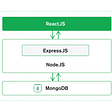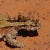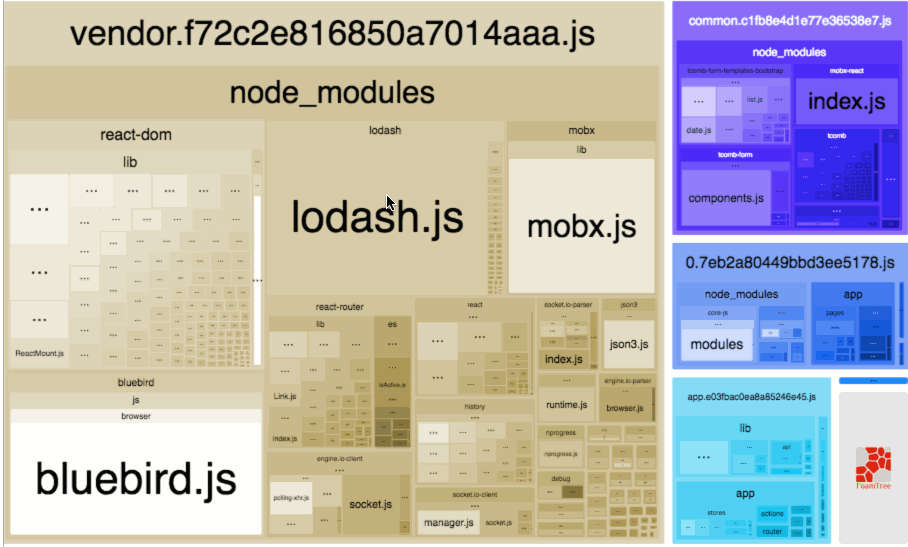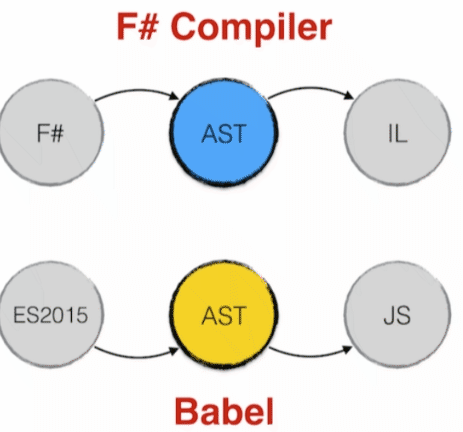# Eight Queens Puzzle in TypeScript’s Type System

## What is the Eight Queens puzzle?

`/* Generate an array containing number belows `n` */function range(n: number): number[] {  return Array(n)    .fill(0)    .map((_, i) => i);}/* Ensure the last queen in the passed array is safe from the other queens.*/function validate(queens: number[]): boolean {  const lastPos = queens.length - 1;  const last = queens[lastPos];  return !range(lastPos).some((pos) => {    const stepVal = queens[pos];    return stepVal == last ||            Math.abs(lastPos - pos) == Math.abs(last - stepVal);  });}function findSolution(queens: number[], step: number, tableSize: number): number[] | false {  if (step === tableSize) {    return queens;  } else {    return range(tableSize)      .map((i) => [...queens, i + 1])      .filter((q) => validate(q))      .reduce<number[] | false>((acc, newQueens) => {        return acc || findSolution(newQueens, step + 1, tableSize);      }, false);  }}console.log(findSolution([], 0, 8)) // display `[1, 5, 8, 6, 3, 7, 2, 4]``

## Natural numbers

`type Z = { kind: 'Z' } // Represents 0type S<T> = { kind: 'S', succ: T } // Represents the successor of the natural number Ttype N0 = Z     // 0type N1 = S<N0> // 1type N2 = S<N1> // 2type N3 = S<N2> // 3type N4 = S<N3> // 4type N5 = S<N4> // 5type N6 = S<N5> // 6type N7 = S<N6> // 7type N8 = S<N7> // 8`

## Subtraction

`// returns n - m,  or 0 if m > nfunction sub(n: number, m: number): number {  /* Obviously we could also write `return n - m`      But the goal is to have something translatable at type level,      and `-` doesn't exist at type level. */  if ( m > 0 ) {    if ( n > 0 ) {      return sub(n -1, m -1);	    } else {      return 0;		    }   } else {    return n;  }}`
`// Returns N - M, returns 0 if M > Ntype Sub<N, M> =   M extends S<infer PM>?N extends S<infer PN>?Sub<PN, PM>:Z:N;`

## Other Nat operators

`type Eq<A, B> = [A] extends [B] ? ([B] extends [A] ? true : false) : false;`
`type GT<A, B> = A extends S<infer PA> ? (B extends S<infer PB> ? GT<PA, PB> : true) : false;`
`type AbsSub<A, B> = GT<A, B> extends true ? Sub<A, B> : Sub<B, A>;`

## Boolean logic

`type Or<A extends boolean, B extends boolean> = A extends true ? true : B;type Not<A extends boolean> = A extends true ? false : true;`

## Implementing range function

`function range(n: number): number[] {  if ( n > 0 ) {    return [...range(n -1), n - 1]  } else {    return [];  }}`
`type RangeTuple<N> = N extends S<infer PN> ? [...RangeTuple<PN>, PN] : [];`
`type Test1 = RangeTuple<Z> // Test1 is []type Test2 = RangeTuple<N3> // Test2 is [Z, N1, N2]`

## Operations on Tuples

`type Size<ARR> = ARR extends [infer HEAD, ...infer TAIL] ? S<Size<TAIL>> : Z;`
`type At<ARR extends unknown[], N> =   ARR extends [infer HEAD, ...infer TAIL]    ? N extends S<infer PN>      ? At<TAIL, PN>      : HEAD    : never;`

## Implementing `validate` function

`stepVal == last || Math.abs(lastPos - pos) == Math.abs(last - stepVal);`
`type ValidateOnePoint<POS, STEP_VAL, LAST_POS, LAST> = Or<  Eq<STEP_VAL, LAST>,  Eq<AbsSub<LAST_POS, POS>, AbsSub<LAST, STEP_VAL>>>;`
`type ValidateOnePointFromArr<POS, LAST_POS, QUEENS extends unknown[]> = ValidateOnePoint<  POS,  At<QUEENS, POS>,  LAST_POS,  At<QUEENS, LAST_POS>>;`
`function some<A>(arr: A[], predicate: (a:A) => boolean): boolean {   if(arr.length > 0) {      const [head, ...tail] = arr;      if (predicate(head)) {            return true;       } else {           return some(tail, predicate)       }   } else {      return false   }}`
`type SomeInvalidPoint<RANGE, QUEENS extends unknown[]> = RANGE extends [infer HEAD, ...infer TAIL]  ? ValidateOnePointFromArr<HEAD, Sub<Size<QUEENS>, N1>, QUEENS> extends true    ? true    : SomeInvalidPoint<TAIL, QUEENS>  : false;`
`type Validate<QUEENS extends unknown[]> = Not<SomeInvalidPoint<RangeTuple<Sub<Size<QUEENS>, N1>>, QUEENS>>;`
`type TestValidate1 = Validate<[N1, N2]> // equivalent to falsetype TestValidate2 = Validate<[N1, N5]> // equivalent to true`

## Implementing `findSolution`

`function filter<A>(arr: A[], predicate: (a:A) => boolean): A[] {  if(arr.length > 0) {      const [head, ...tail] = arr;      if(predicate(head)) {            return [head, ...filter(tail, predicate)];       } else {           return filter(tail, predicate);       }   } else {      return false;   }}function map<A, B>(arr: A[], fn: (a:A) => B): B[] {  if(arr.length > 0) {    const [head, ...tail] = arr;     return [fn(head), ...map(tail, fn)];   } else {    return [];  }}function reduce<A,B>(arr: A[], fn: (b:B, a:A) => B, init: B): B {  if (arr.length > 0) {    const [head, ...tail] = arr;    return reduce(tail, fn, fn(init, head));	  } else {    return init;  }}`
`type FilterValidArr<QUEENS extends unknown[][]> = QUEENS extends [infer HEAD extends unknown[], ...infer TAIL extends unknown[][]]      ? Validate<HEAD> extends true        ? [HEAD, ...FilterValidArr<TAIL>]        : FilterValidArr<TAIL>  : [];type MapPossiblePositions<QUEENS extends unknown[], TABLE_SIZE> = TABLE_SIZE extends S<infer PN>  ? [...MapPossiblePositions<QUEENS, PN>, [...QUEENS, TABLE_SIZE]]  : [];type ReduceToOneSolution<INIT, ARR, STEP, TABLE_SIZE> = ARR extends [infer HEAD extends unknown[], ...infer TAIL]    ? ReduceToOneSolution<        INIT extends false ? FindSolution<HEAD, S<STEP>, TABLE_SIZE> : INIT,        TAIL,        STEP,        TABLE_SIZE      >  : INIT;`
`type FindSolution<QUEENS extends unknown[], STEP, TABLE_SIZE> = Eq<STEP, TABLE_SIZE> extends true  ? QUEENS  : ReduceToOneSolution<false, FilterValidArr<MapPossiblePositions<QUEENS, TABLE_SIZE>>, STEP, TABLE_SIZE>;`

## Checking the result

`type Result = FindSolution<[], Z, N8>`

--

--

--

## More from Benoit Lemoine

I’m a full-stack developer, in love with functional programming and type systems. I’m working currently at Decathlon Canada, in Montreal QC.

Love podcasts or audiobooks? Learn on the go with our new app.

## Send multiple requests for data with axios.all( )## Shopify App With Laravel, InertiaJs, and Polaris## React side bar (but sexy?)## MERN Stack## Tutorial: User Authentication with Passport.js## Benoit Lemoine

I’m a full-stack developer, in love with functional programming and type systems. I’m working currently at Decathlon Canada, in Montreal QC.

## Rush to Retire NPM for RushJS## Analyze and Optimize Webpack Bundles Size and Contents## From Apollo to Urql — Part 2## TypeScript and ML languages